# The 5,10-Duoprism

The 5,10-duoprism is a 4D uniform polychoron in the infinite duoprism family. It is the Cartesian product of a decagon and a pentagon, and has a surface consisting of 15 cells (10 pentagonal prisms and 5 decagonal prisms), 65 polygons (5 decagons, 10 pentagons, and 50 squares), 100 edges, and 50 vertices.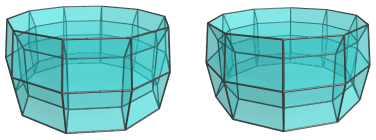The 5,10-duoprism can be augmented with pentagonal prism pyramids and remain convex. The dichoral angle between two adjacent pentagonal prisms, which is the internal angle of the regular decagon, is exactly 144°; and the dichoral angle between the base pentagonal prism and a pentagonal pyramid cell in the pentagonal prism pyramid is exactly 18°. Thus, the pentagonal pyramid cells from two adjacent augments would merge into a pentagonal bipyramid (J13). Augmenting all the pentagonal prisms with pentagonal prism pyramids therefore produces the deca-augmented 5,10-duoprism, which sports a ring of 10 pentagonal bipyramids.

## Structure

### Centered on Ring of Decagonal Prisms

The 5,10-duoprism consists of a ring of 10 pentagonal prisms: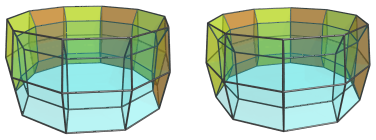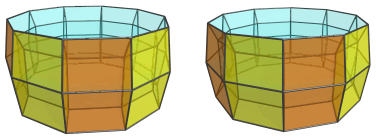These pentagonal prisms lie at a sharp angle to the 4D viewpoint, and therefore appear quite flattened by foreshortening. In 4D, of course, they are perfectly uniform, undistorted pentagonal prisms.

This ring of cells mutually wraps around a ring of 5 decagonal prisms: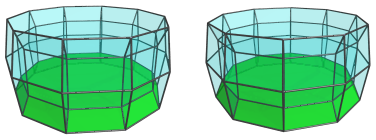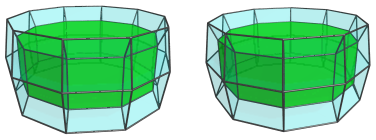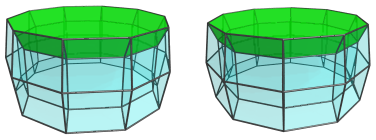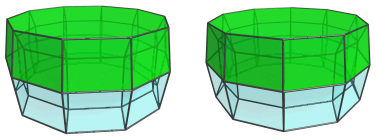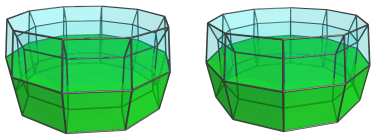### Centered on Ring of Pentagonal Prisms

Now we take a look at the 5,10-duoprism from a different viewpoint, this time centered on the ring of pentagonal prisms. Again, there are 10 pentagonal prisms wrapped around in a ring: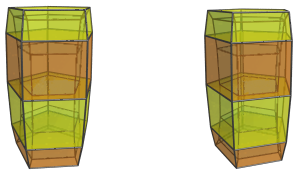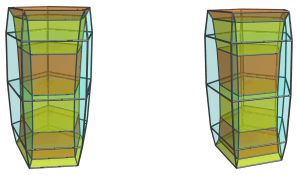Wrapping around these cells is the ring of 5 decagonal prisms: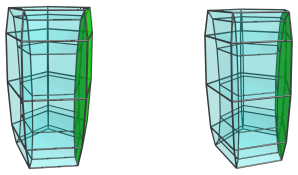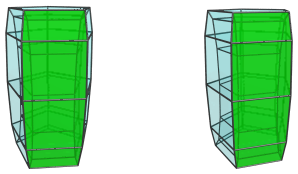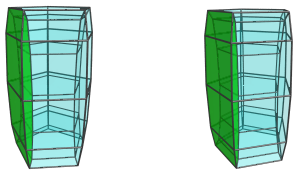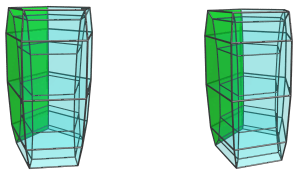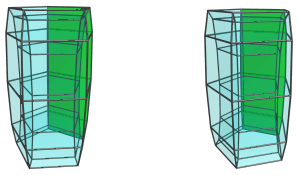## Coordinates

The Cartesian coordinates of the 5,10-duoprism, centered on the origin and with edge length 2, are:

• (A, 0, ±2φ, 0)
• (B, ±φ, ±2φ, 0)
• (C, ±1, ±2φ, 0)
• (A, 0, ±1, ±D)
• (B, ±φ, ±1, ±D)
• (C, ±1, ±1, ±D)
• (A, 0, ±φ2, ±E)
• (B, ±φ, ±φ2, ±E)
• (C, ±1, ±φ2, ±E)

where:

 A = √((10+2√5)/5) B = √((5−√5)/10) C = −√((5+2√5)/5) D = √(3+4φ) E = √(2+φ)

and φ=(1+√5)/2 is the Golden Ratio.

Last updated 05 Apr 2019.